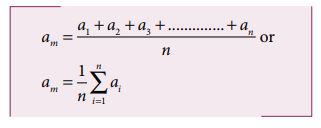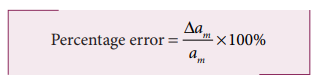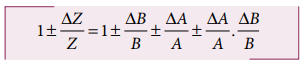Home | | Physics 11th std | Theory of Errors

# Theory of Errors

The foundation of all experimental science and technology is measurement.

THEORY OF ERRORS

The foundation of all experimental science and technology is measurement. The result obtained from any measurement will contain some uncertainty. Such an uncertainty is termed error. Any calculation made using the measured values will also have an error. It is not possible to make exact measurements in an experiment. In measurements, two different terms accuracy and precision are used and need to be distinguished at this stage. Accuracy refers to how far we are from the true value, and precision refers to how well we measure.

## Accuracy and Precision

Let us say, you know your true height is exactly 59. You first measure your height with a yardstick and get the value 50. Your measurement is hence not accurate. Now you measure your height with a laser yardstick and get 59 as the value. Now your measurement is accurate. The true value is also called theoretical value. The level of accuracy required for each application varies greatly. Highly accurate data can be very difficult to produce and compile. For example, if you consistently measure your height as 50 with a yard stick, your measurements are precise. The level of precision required for different applications vary to a great extent. Engineering projects such as road and utility construction require very precise information measured to the millimeter or one-tenth of an inch.

If a measurement is precise, that does not necessarily mean that it is accurate. However, if the measurement is consistently accurate, it is also precise.

For example, if the temperature outside a building is 40oC as measured by a weather thermometer and if the real outside temperature is 40oC, the thermometer is accurate. If the thermometer consistently registers this exact temperature in a row, the thermometer is precise.

Consider another example. Let the temperature of a refrigerator repeatedly measured by a thermometer be given as 10.4oC, 10.2oC, 10.3oC, 10.1oC, 10.2oC, 10.1oC, 10.1oC, 10.1oC. However, if the real temperature inside the refrigerator is 9oC, we say that the thermometer is not accurate (it is almost one degree off the true value), but since all the measured values are close to 10oC, hence it is precise.

## A visual example:

Target shooting is an example which explains the difference between accuracy and precision. In Figure 1.9 (a), the shots are focused so as to reach the bull’s eye (midpoint), but the arrows have reached only around this point. Hence the shots are not accurate and also not precise.In Figure 1.9 (b), all the shots are close to each other but not at the central point. Hence the shots are said to be precise but not accurate. In Figure 1.9 (c), the shots are closer and also at the central point. Hence the shots are both precise and accurate.

## A numerical example

The true value of a certain length is near 5.678 cm. In one experiment, using a measuring instrument of resolution 0.1 cm, the measured value is found to be 5.5 cm. In another experiment using a measuring instrument of greater resolution, say 0.01 cm, the length is found to be 5.38 cm. We find that the first measurement is more accurate as it is closer to the true value, but it has lesser precision. On the contrary, the second measurement is less accurate, but it is more precise.

## Errors in Measurement

The uncertainty in a measurement is called an error. Random error, systematic error and gross error are the three possible errors.

### i) Systematic errors

Systematic errors are reproducible inaccuracies that are consistently in the same direction. These occur often due to a problem that persists throughout the experiment. Systematic errors can be classified as follows

#### a. Instrumental errors

When an instrument is not calibrated properly at the time of manufacture, instrumental errors may arise. If a measurement is made with a meter scale whose end is worn out, the result obtained will have errors. These errors can be corrected by choosing the instrument carefully.

#### b. Imperfections in experimentaltechnique or procedure

These errors arise due to the limitations in the experimental arrangement. As an example, while performing experiments with a calorimeter, if there is no proper insulation, there will be radiation losses. This results in errors and to overcome these, necessary correction has to be applied.

#### c. Personal errors

These errors are due to individuals performing the experiment, may be due to incorrect initial setting up of the experiment or carelessness of the individual making the observation due to improper precautions.

#### d. Errors due to external causes

The change in the external conditions during an experiment can cause error in measurement. For example, changes in temperature, humidity, or pressure during measurements may affect the result of the measurement.

#### e. Least count error

Least count is the smallest value that can be measured by the measuring instrument, and the error due to this measurement is least count error. The instrument’s resolution hence is the cause of this error. Least count error can be reduced by using a high precision instrument for the measurement.

### ii. Random errors

Random errors may arise due to random and unpredictable variations in experimental conditions like pressure, temperature, voltage supply etc. Errors may also be due to personal errors by the observer who performs the experiment. Random errors are sometimes called “chance error”. When different readings are obtained by a person every time he repeats the experiment, personal error occurs. For example, consider the case of the thickness of a wire measured using a screw gauge. The readings taken may be different for different trials. In this case, a large number of measurements are made and then the arithmetic mean is taken.

If n number of trial readings are taken in an experiment, and the readings are a1, a2, a3,…………………. an. The arithmetic mean isUsually this arithmetic mean is taken as the best possible true value of the quantity.

Certain procedures to be followed to minimize experimental errors, along with examples are shown in Table 1.8.### iii. Gross Error

The error caused due to the shear carelessness of an observer is called gross error.

For example

i. Reading an instrument without setting it properly.

ii. Taking observations in a wrong manner without bothering about the sources of errors and the precautions.

iii. Recording wrong observations.

iv. Using wrong values of the observations in calculations.

These errors can be minimized only when an observer is careful and mentally alert.

## Error Analysis

### i. Absolute Error

The difference between the true value and the measured value of a quantity is called absolute error. If a1, a2, a3, ………. an are the measured values of any quantity ‘a’ in an experiment performed n times, then the arithmetic mean of these values is called the true value (am) of the quantity.The absolute error in measured values is given by### ii. Mean Absolute error

The arithmetic mean of the magnitude of absolute errors in all the measurements is called the mean absolute error.If am is the true value and am is the mean absolute error then the magnitude of the quantity may lie between am + am and am -am

### iii. Relative error

The ratio of the mean absolute error to the mean value is called relative error. This is also called as fractional error (or) relative error.Thus Relative error expresses how large the absolute error is compared to the total size of the object measured. For example, a driver’s speedometer shows that his car is travelling at 60 km h-1 when it is actually moving at 62 kmh-1. Then absolute error of speedometer is 62-60 km h-1 = 2 km h-1 Relative error of the measurement is 2 km h-1 / 60 km h-1 = 0.033.

### iv. Percentage error

The relative error expressed as a percentage is called percentage error.A percentage error very close to zero means one is close to the targeted value, which is good and acceptable. It is always necessary to understand whether error is due to impression of equipment used or a mistake in the experimentation.

## Propagation of errors

A number of measured quantities may be involved in the final calculation of an experiment. Different types of instruments might have been used for taking readings. Then we may have to look at the errors in measuring various quantities, collectively.

The error in the final result depends on

i. The errors in the individual measurements

ii. On the nature of mathematical operations performed to get the final result. So we should know the rules to combine the errors.

The various possibilities of the propagation or combination of errors in different mathematical operations are discussed below:

### i. Error in the sum of two quantities

Let ∆A and ∆B be the absolute errors in the two quantities A and B respectively. Then,

Measured value of A = A ± ∆A

Measured value of B = B ± ∆B

Consider the sum, Z = A + B

The error ∆Z in Z is then given by### ii. Error in the difference of two quantities

Let ΔA and ΔB be the absolute errors in the two quantities, A and B, respectively. Then,

Measured value of A = A ± ΔA

Measured value of B = B ± ΔB

Consider the difference, Z = A – B

The error ΔZ in Z is then given by Z ± ΔZ = (A ± ΔA) – (B ± ΔB)### (iii) Error in the product of two quantities

Let ΔA and ΔB be the absolute errors in the two quantities A, and B, respectively. Consider the product Z = AB

The error ΔZ in Z is given by Z ± ΔZ = (A ± ΔA) (B ± ΔB)Dividing L.H.S by Z and R.H.S by AB, we get,As ΔA /A, ΔB / B are both small quantities, their product term ΔA/A . ΔB/B can be neglected. The maximum fractional error in Z is### (iv) Error in the division or quotient of two quantities

Let ΔA and ΔB be the absolute errors in the two quantities A and B respectively.As the terms ΔA/A and ΔB/B are small, their product term can be neglected.### (v) Error in the power of a quantity

Consider the nth power of A, Z = An The error ΔZ in Z is given byWe get [(1+x)n ≈1+nx, when x<<1] neglecting remaining terms, Dividing both sides by ZTags : Accuracy and Precision, Errors in Measurement, Error Analysis, Propagation of errors , 11th Physics : UNIT 1 : Nature of Physical World and Measurement
Study Material, Lecturing Notes, Assignment, Reference, Wiki description explanation, brief detail
11th Physics : UNIT 1 : Nature of Physical World and Measurement : Theory of Errors | Accuracy and Precision, Errors in Measurement, Error Analysis, Propagation of errors